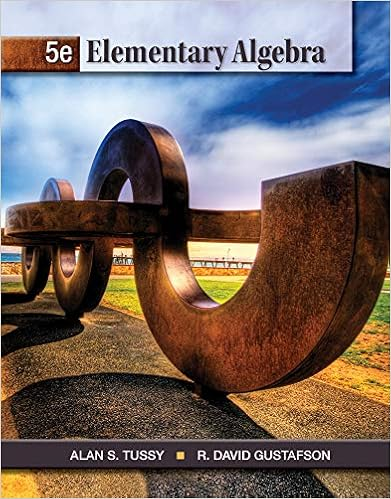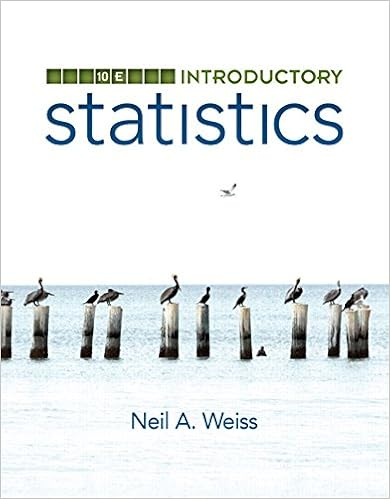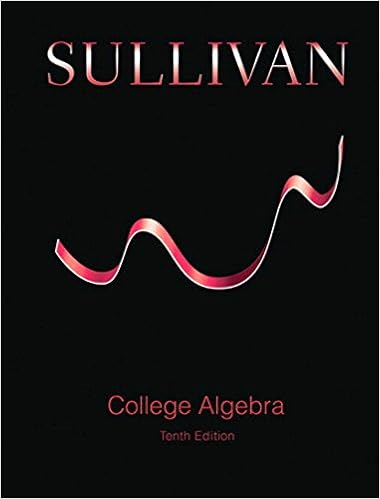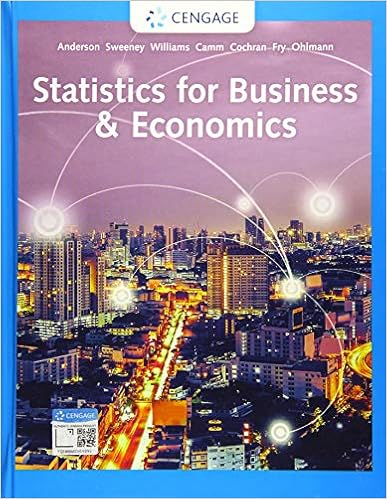# 1 u v is in v 2 u v v u 3 u v w u v w 4 there is a 0

• Notes
• 20

This preview shows page 8 - 10 out of 20 pages.

1.u + v is in V2.u + v = v + u3.(u + v) + w = u + (v + w)4.There is a 0 vector in V such that u + 0 = ua.Note: the zero vector is different in each vector space. For example, a vector V can be in R3 or R2. they aretwo different zero vectors5.u + (-u) = 06.cu is in V7.c(u + v) = cu + cv8.(c + d) u = cu + du9.c(du) = (cd)u10.1u = u-Rn, where n >= 1, is a good EXAMPLE of vector spaces. Geometrically think about R3 to help you visualize the otherconcepts of vector spaces.-Polynomials are also vector spaces because they follow the axioms. Multiplying a polynomial by a scalar gives you apolynomial. Adding two polynomials together gives you a polynomial.-So far we have extended many of the properties of R2 and R3 to Rn . We don’t have to stop there. By taking the mostfundamental properties of these “natural” sets of vectors, we can define a general vector space. Any set of objectsequipped with an addition and scalar multiplication which satisfies the eight vector space axioms qualifies as a vectorspace.-Basically, we know about the geometric Rn space.-But anything can be a vector space (doesn’t need to be explicitly a coordinate system) as long as it is closed underaddition and multiplication.SUBSPACESTo check if a space H is a subspace of vector space V, you have to check for 3 of the 10 above axioms. The rest are automaticallysatisfied.a.The zero vector of V is in Hb.H is closed under vector additionc.H is closed under multiplication by scalars.-Every vector space is a subspace of some larger vector space.
##### We have textbook solutions for you!The document you are viewing contains questions related to this textbook.
Chapter 2 / Exercise 124
Elementary Algebra
Tussy/GustafsonExpert VerifiedBrowse all Textbook Solutions
-Every subspace is a vector space-Sayingsubspace of Vlets you know that V is the lager vector space.A SUBSPACE SPANNED BY A SET-One of the most common ways of describing a subspace is by spans.-Recall: a span is the space of the possible linear combinations of vectorsTheorem 1:If v1...vp are in a vector space V, then Span{v1...vp} is a subspace of V.-We call Span{v1...vp} the subspace spanned (or generated) by {v1….vp}-This is because a span is basically scaling and adding the vectors together. And a vector space is closed under addition andmultiplication by scalar. So naturally, the span is also in the vector space.Questions I may be asked-For what values of h will y be in the subspace of R3 spanned by v1, v2, v3. You will be given v1...v3. And y will have h as amissing variable. So, basically find the value of h so that the vector y is in the subspace of R3-To do so, you are checking to see if y is in the span of v1...v3.-Solve this like you solved other problems. Check if y is a linear combination of v1...v3.-So put v1...v3 in a matrix and the y vector as the augmented last column.-Solve and see what you need h to be.If asked how you know that a span of a certain vectors is a subspace of Rn, say →-You know it is a subspace of Rn because of Theorem 1-This states that a span of vectors in Rn is a subspace of that Rn4.2 Null Spaces, Column Spaces, and Linear TransformationsNull and Column spaces are both vector subspaces that are associated with matrices. (they mark the solution sets in V that solve yourparticular matrices)-

Course Hero member to access this document

Course Hero member to access this document

End of preview. Want to read all 20 pages?

Course Hero member to access this document

Term
Spring
Professor
Chorin
##### We have textbook solutions for you!
The document you are viewing contains questions related to this textbook.The document you are viewing contains questions related to this textbook.
Chapter 2 / Exercise 124
Elementary Algebra
Tussy/GustafsonExpert Verified
•••